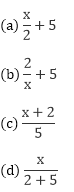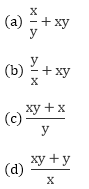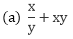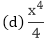# Chapter 8: Introduction to Algebra – Exercise 8.3

### Question: 1

5 more than twice a number x is written as:

(a) 5 + x + 2

(b) 2x + 5

(c) 2x – 5

(d) 5x + 2

(b) 2x + 5

### Question: 2

The quotient of x by 2 is written as:### Solution:### Question: 3

The quotient of x by 3 is multiplied by y is written as:

(a)  x/3y

(b)  3x/y

(c)  3y/x

(d)  xy/3

(d)  xy/3

### Question: 4

9 taken away from the sum of x and y is

(a) x + y – 9

(b) 9 – (x + y)(a) x + y – 9

### Question: 5

The quotient of x by y added to the product of x and y is written as:### Solution:### Question: 6

a2b3 × 2ab2 is equal to

(a) 2a3b4

(b) 2a3b5

(c) 2ab

(d) a3b5

(b) 2a3b5

### Question: 7

4a2b3 × 3ab2 × 5a3b is equal to

(a) 60a3b5

(b) 60a6b5

(c) 60a6b6

(d) a6b6

(c) 60a6b6

### Question: 8

If 2x2y and 3xy2 denote the length and breadth of a rectangle, then its area is

(a) 6xy

(b) 6x2y2

(c) 6x3y3

(d) x3y3

(c) 6x3y3

### Question: 9

In a room there are x2 rows of chairs and each rows contains 2x2 chairs. The total number of chairs in the room is

(a) 2x2

(b) 2x4

(c) x4(b) 2x4

### Question: 10

a3 × 2a2b × 3ab5 is equal to

(a) a6b6

(b) 23a6b6

(c) 6a6b6

(d) None of these

(b) 2x4